# But if you divide or multiply by a negative – Flip

If you multiply or divide by a negative you must

reverse the inequality sign
You must FLIP IT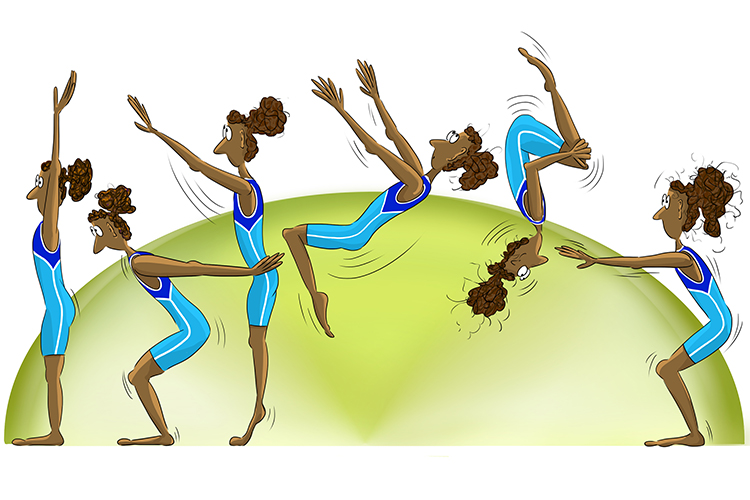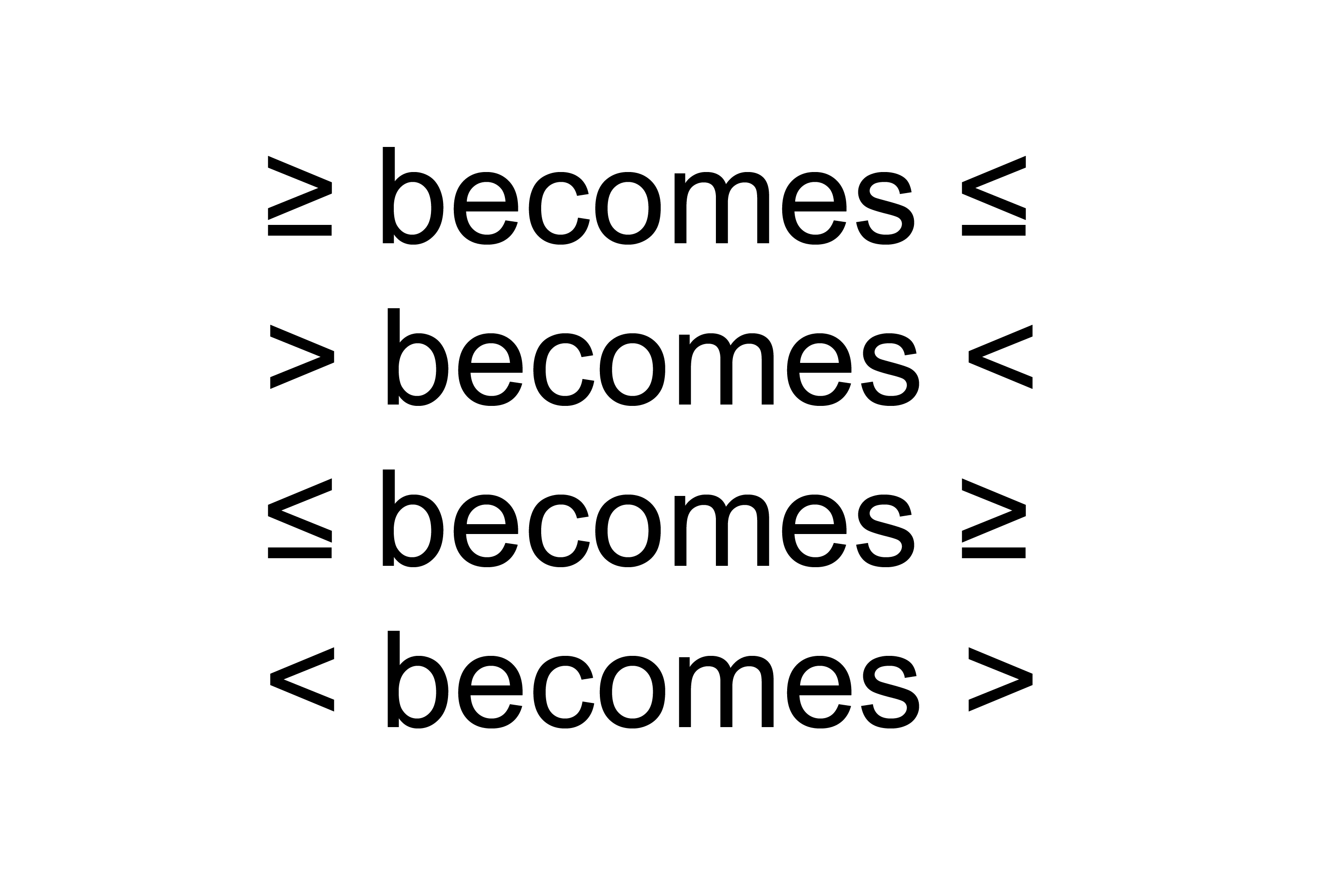Why

Just try it on a number you know and then you will understand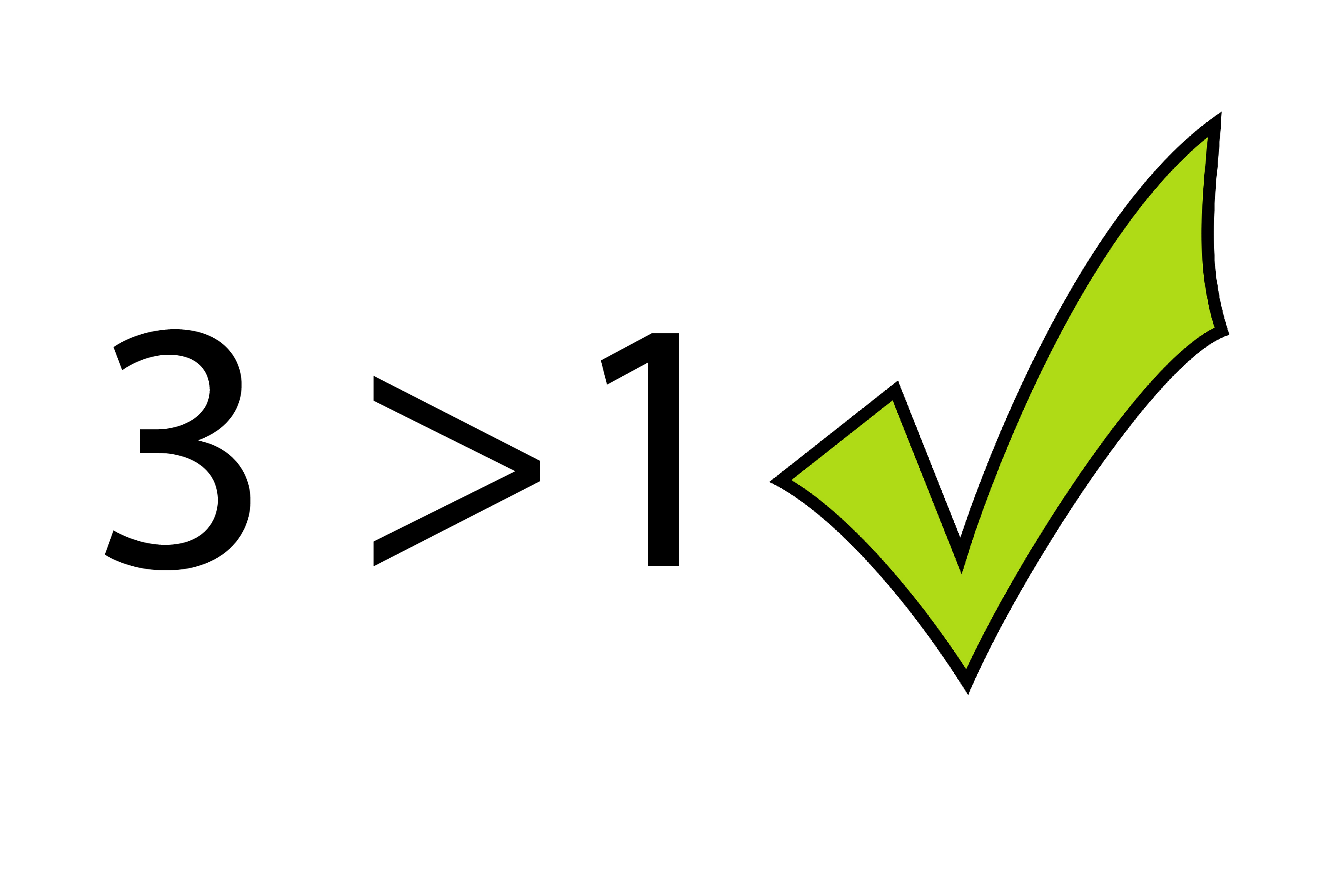3  is greater than 1

Divide by -1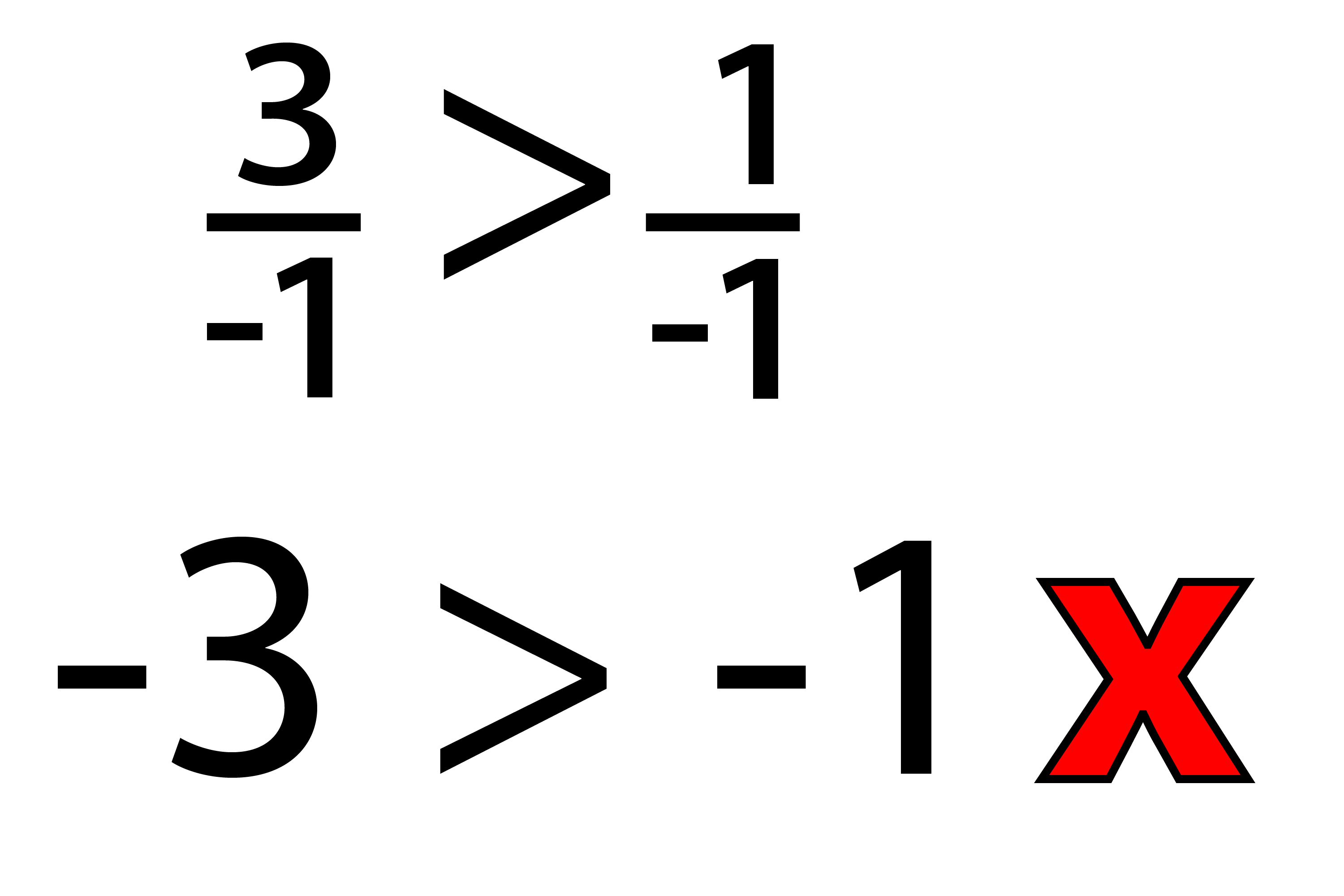-3 is NOT greater than -1You MUST FLIP/REVERSE the inequality sign.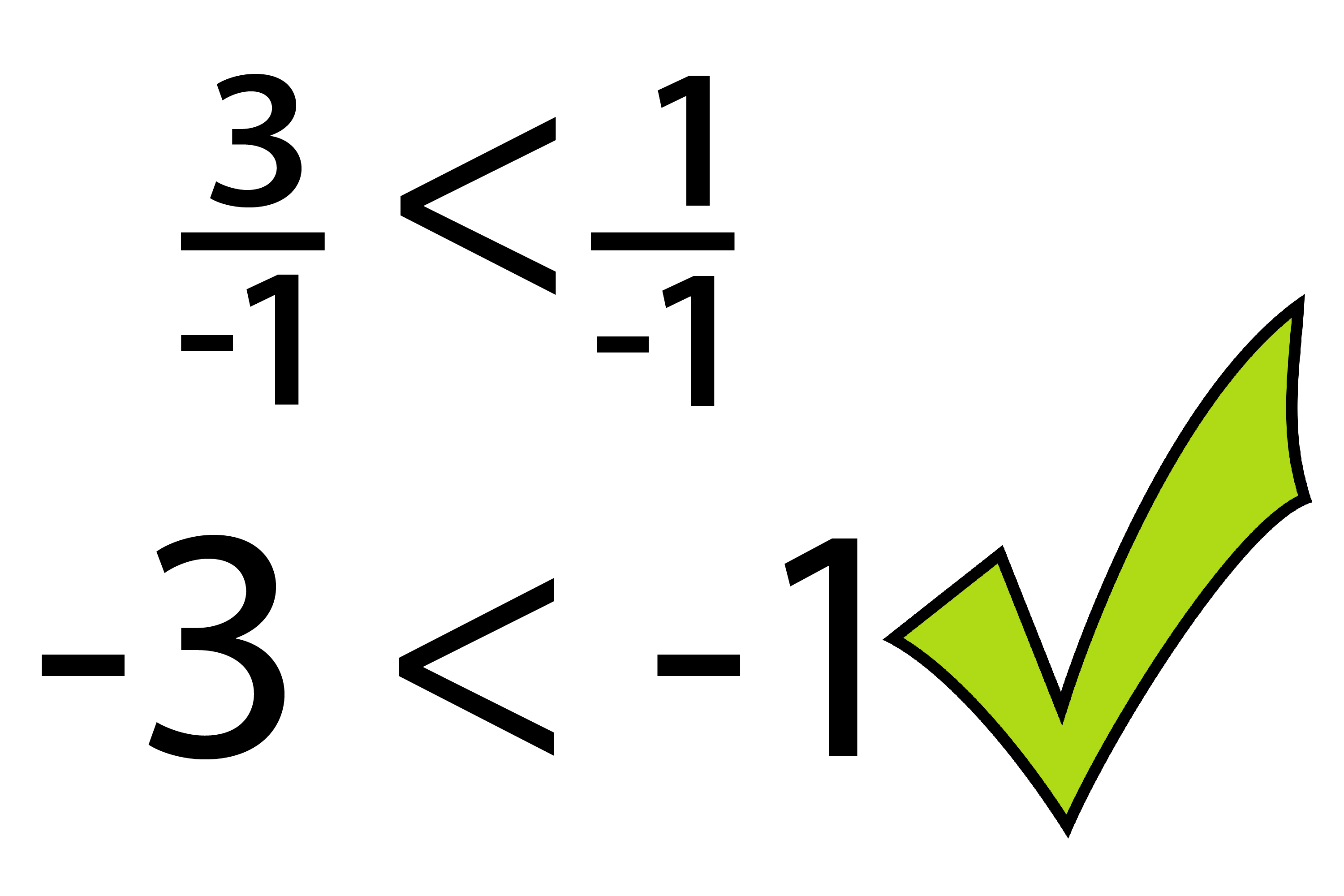-3 is less than -1